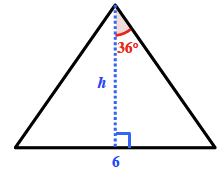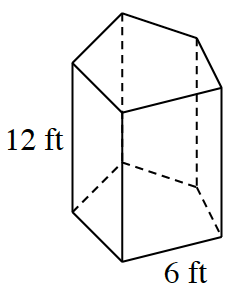### Home > CCG > Chapter Ch10 > Lesson 10.1.4 > Problem10-46

10-46.

Calculate the total surface area and volume of the prism at right. Assume that the base is a regular pentagon.

Find the central angle of the pentagon.

$\frac{360º}{5}=72º$

The area of the triangle will help you find the area of the pentagon.$\text{tan}(36^º)=\frac{3}{h}$$h=\frac{3}{\text{tan}(36^º)}$, $h\approx4.1291\text{ ft}$

Area of the triangle $= (0.5)(4.1291)(6) ≈ 12.39\text{ ft}²$
Area of pentagon $= (5)(12.39) ≈ 61.9\text{ ft}²$

Find the area of each of the sides, and sum them to find the surface area.
$(12)(6)(5) = 360$
$360 + 2(61.9) ≈ 483.8\text{ ft}²$

Multiply the area of the pentagon by the height to find the volume.
$(61.9)(12) ≈ 743.3\text{ ft}³$

Volume $≈ 743.3\text{ ft}³$
Surface area $≈ 483.3\text{ ft}²$